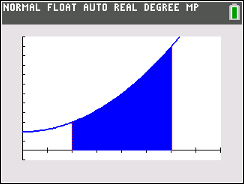Activities

•• Subject Area

• Math: Calculus: Definite Integrals and Applications

9-12

50 Minutes

• Device
•TI-84 Plus CE
•TI-84 Plus C Silver Edition

Bounded AreasActivity Overview

In this TI-84 family lesson, students will compare the Riemann Sum area estimates with the exact bounded area of a curve and the x-axis.

Objectives

• Students will practice and discuss applications of finding the area bounded by a curve and the x-axis.
• Students will apply this information to finding the area bounded by two curves.
• Students will try to make a connection with how to understand these topics in both IB Mathematics and AP Calculus and on their final assessments.

Vocabulary

• Riemann Sums
• Underestimate
• Overestimate
• Definite Integrals
• Bounded Areas
• Concavity